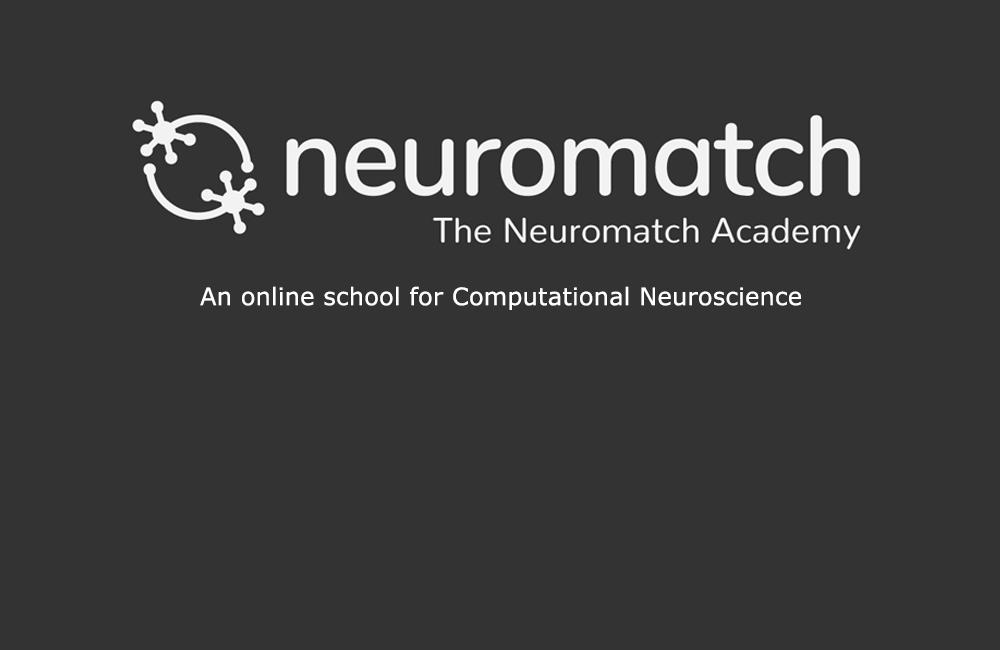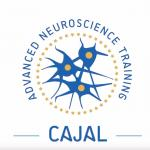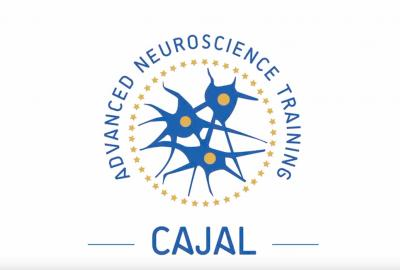# Model Fitting

Category
Level
Beginner

Neuromatch Academy aims to introduce traditional and emerging tools of computational neuroscience to trainees. It is appropriate for student population ranging from undergraduates to faculty in academic settings and also includes industry professionals. In addition to teaching the technical details of computational methods, Neuromatch Academy also provide a curriculum centered on modern neuroscience concepts taught by leading professors along with explicit instruction on how and why to apply models.

This course focuses on the purpose of model fitting, approaches to model fitting, model fitting for linear models, and how to assess the quality and compare model fits, as well as a 10-step practical guide on how to succeed in modeling.

Course Features
Lectures
Interactive Tutorials
Recordings of question and answer sessions
Discussion Forum on Neurostars.org
Lessons of this Course
1
1
Duration:
26:46

This lecture is part of the Neuromatch Academy (NMA), a massive, interactive online summer school held in 2020 that provided participants with experiences spanning from hands-on modeling experience to meta-science interpretation skills across just about everything that could reasonably be included in the label "computational neuroscience".

This lecture focuses on the purpose of model fitting, approaches to model fitting, model fitting for linear models, and how to assess the quality and compare model fits. We will present a 10-step practical guide on how to succeed in modeling.

2
2
Duration:
6:18
Speaker:

This is Tutorial 1 of a series on fitting models to data. We start with simple linear regression, using least squares optimization (Tutorial 1) and Maximum Likelihood Estimation (Tutorial 2). We will use bootstrapping to build confidence intervals around the inferred linear model parameters (Tutorial 3). We'll finish our exploration of regression models by generalizing to multiple linear regression and polynomial regression (Tutorial 4). We end by learning how to choose between these various models. We discuss the bias-variance trade-off (Tutorial 5) and Cross Validation for model selection (Tutorial 6).

3
3
Duration:
8:00
Speaker:

This is Tutorial 2 of a series on fitting models to data. We start with simple linear regression, using least squares optimization (Tutorial 1) and Maximum Likelihood Estimation (Tutorial 2). We will use bootstrapping to build confidence intervals around the inferred linear model parameters (Tutorial 3). We'll finish our exploration of regression models by generalizing to multiple linear regression and polynomial regression (Tutorial 4). We end by learning how to choose between these various models. We discuss the bias-variance trade-off (Tutorial 5) and Cross Validation for model selection (Tutorial 6).

In this tutorial, we will use a different approach to fit linear models that incorporates the random 'noise' in our data.

4
4
Duration:
5:00
Speaker:

This is Tutorial 3 of a series on fitting models to data. We start with simple linear regression, using least squares optimization (Tutorial 1) and Maximum Likelihood Estimation (Tutorial 2). We will use bootstrapping to build confidence intervals around the inferred linear model parameters (Tutorial 3). We'll finish our exploration of regression models by generalizing to multiple linear regression and polynomial regression (Tutorial 4). We end by learning how to choose between these various models. We discuss the bias-variance trade-off (Tutorial 5) and Cross Validation for model selection (Tutorial 6).

In this tutorial, we will discuss how to gauge how good our estimated model parameters are.

5
5
Duration:
7:50
Speaker:

This is Tutorial 4 of a series on fitting models to data. We start with simple linear regression, using least squares optimization (Tutorial 1) and Maximum Likelihood Estimation (Tutorial 2). We will use bootstrapping to build confidence intervals around the inferred linear model parameters (Tutorial 3). We'll finish our exploration of regression models by generalizing to multiple linear regression and polynomial regression (Tutorial 4). We end by learning how to choose between these various models. We discuss the bias-variance trade-off (Tutorial 5) and Cross Validation for model selection (Tutorial 6).

In this tutorial, we will generalize the regression model to incorporate multiple features.

6
6
Duration:
6:38
Speaker:

This is Tutorial 5 of a series on fitting models to data. We start with simple linear regression, using least squares optimization (Tutorial 1) and Maximum Likelihood Estimation (Tutorial 2). We will use bootstrapping to build confidence intervals around the inferred linear model parameters (Tutorial 3). We'll finish our exploration of regression models by generalizing to multiple linear regression and polynomial regression (Tutorial 4). We end by learning how to choose between these various models. We discuss the bias-variance trade-off (Tutorial 5) and Cross Validation for model selection (Tutorial 6).

In this tutorial, we will learn about the bias-variance tradeoff and see it in action using polynomial regression models.

7
7
Duration:
5:28
Speaker:

This is Tutorial 6 of a series on fitting models to data. We start with simple linear regression, using least squares optimization (Tutorial 1) and Maximum Likelihood Estimation (Tutorial 2). We will use bootstrapping to build confidence intervals around the inferred linear model parameters (Tutorial 3). We'll finish our exploration of regression models by generalizing to multiple linear regression and polynomial regression (Tutorial 4). We end by learning how to choose between these various models. We discuss the bias-variance trade-off (Tutorial 5) and Cross Validation for model selection (Tutorial 6).

8
8
Duration:
38.17
Speaker:

This lecture is part of the Neuromatch Academy (NMA), a massive, interactive online summer school held in 2020 that provided participants with experiences spanning from hands-on modeling experience to meta-science interpretation skills across just about everything that could reasonably be included in the label "computational neuroscience".

This lecture summarizes the concepts introduced in Model Fitting I and adds two additional concepts: 1) MLE is a frequentist way of looking at the data and the model, with its own limitations. 2) Side-by-side comparisons of bootstrapping and cross-validation.

##### Recent courses#### Cajal Course in Computational Neuroscience#### Deep learning: Graphs